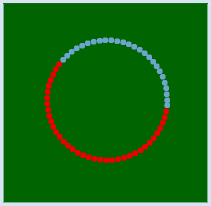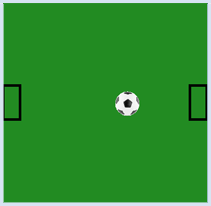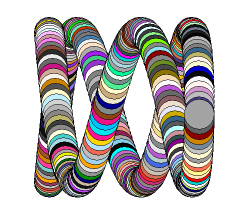deutsch     english    français     Print

## 3.5 GLOBAL VARIABLES, ANIMATIONS### INTRODUCTION

 Computer graphics are frequently used to represent time-varying content. For example, you can simulate a process from physics or biology, or create a computer game. We generally call such a program an animation. In order to show a temporal sequence, new images are drawn one after another, always after an equal step in time, called an animation step. PROGRAMMING CONCEPTS: Global variables, side effects, double buffering

### CHANGING GLOBAL VARIABLES

 You want to illustrate a ball that moves on a circle. You will get a circular motion with a radius of 1 by calculating the x-coordinate using the cosine function with an increasing parameter t (corresponding to advancing time), and the y-coordinate with the sine function, thus x = cos(t) and y = sin(t).  If you want a different radius, you have to multiply both values with the radius. With the function step(), the situation for each animation step is drawn. Once the ball has made a full circle, the color should change.It is common to introduce an endless animation loop in the main program that repeatedly calls step(). By incorporating a delay, you can change the speed of the animation. When using step(), the global variable t should increase at each step and reset to 0 once it has reached 2P, and the color should change.

```import math
from gpanel import *

def step():
global t
x = r * math.cos(t)
y = r * math.sin(t)
t = t + 0.1
if t > 6.28:
t = 0
setColor(getRandomX11Color())
move(x, y)
fillCircle(10)

makeGPanel(-500, 500, -500, 500)
bgColor("darkgreen")

t = 0
r = 200

while True:
step()
delay(10)
```
Highlight program code (Ctrl+C copy, Ctrl+V paste)

### MEMO

 Python prohibits changing the value of global variables in functions. We can bypass this by providing the variable with the keyword global in the function. The identifier global poses risks: any function can not only change a variable designated as global, but can also create it, as the following example shows: ```def set(): global a a = 2 def get(): print "a =", a set() get() ``` Since set() generates a variable a which is visible throughout the entire program, we also say that the function set() has side effects. Also note how nicely several things, one after another, can be written into the console using comma separation in a print statement. In the output the comma is replaced by a space.

### THE TRICK WITH THE EVEN TICK

The animation loops should run in time ticks that are as even as possible, i.e. with the desired animation period, otherwise the movement will be jerky. With step() each new animation state is set up. Depending on the situation, this can take different amounts of time to complete, as the program may not always run the same parts of the code, and also because the computer may be busy with other tasks in the background, which may delay the execution of the Python code. To compensate for the step() of varying length, the following trick is used, which you could also have figured out yourself: before calling step(), you keep track of the current time using the variable startTime. After returning from step() you wait in a waiting loop until the difference between the new time and the start time reaches the animation period.

 The program moves a soccer ball from goal to goal. For this, you use an image football.gif hat is located in the directory sprites of the TigerJython distribution. You can also take your own picture by copying the file into an appropriate directory on your computer and passing the file path as a parameter in image() (absolute or relative to the directory where your program is located).```from gpanel import *
import time

def step():
global x
global v
clear()
lineWidth(5)
move(25, 300)
rectangle(50, 100)
move(575, 300)
rectangle(50, 100)
x = x + v
image("_sprites/football.gif", x, 275)
if x > 500 or x < 50:
v = -v

makeGPanel(0, 600, 0, 600)
bgColor("forestgreen")
enableRepaint(False)

x = 300
v = 10

while True:
startTime = time.clock()
step()
repaint()
while (time.clock() - startTime)  < 0.020:
delay(1)
```
Highlight program code (Ctrl+C copy, Ctrl+V paste)

### MEMO

 Using time.clock() you can get the current time as a decimal number. The given value is dependent on the computer (processor time, or the time since the first call of clock()). But since you only need the time difference, it does not matter. Save the time before calling step() and wait at the end of the animation loop with a delay(1), until the difference between the current time and the start time reaches the animation period (in seconds). Remember this trick, for you will use it for many processes that ought to run as periodically as possible. Every graphics command is immediately visible in the GPanel window. Deleting with clear() while animating will briefly show an empty graphics window, which can lead to a flickering effect. To avoid this, double buffering should be used in animations. You can achieve this using the command enableRepaint(False), causing the graphics command to be executed only in a background buffer (off screen buffer) and effects are no longer visible. So clear() only deletes the background buffer and does not erase the graphics window anymore. You must trigger the display of the graphics buffers on the screen (called rendering) yourself at the right moment by calling  repaint(). In this program you also need to globally distinguish the variables x and v in the function step() since they are changed in the function.

### EXERCISES

1.

If you do not move the x- and y-coordinates with ordinary cosine or sine functions as you did in your first program, but rather at different rates, it will create interesting curve patterns called Lissajoux figures. Draw such figures with a resolution of 1/1000 in the range of t = 0 to 2π  and with

x = cos(4.5 * t) und y = sin(6.3 * t)

2.

Instead of using fixed numbers, use the variables omega_x and omega_y to draw the figure for the following values:

 omega_x omega_y 3 5 3 7 5 7

Do you see a connection between the figure and the values of omega_x and omega_y?

3.

Draw the Lissajoux figure with omega_x = 2 and omega_y = 7, in the range of t = 0 to 2π, and with a resolution of 1/100 in a GPanel with the coordinates -2 to 2 (both axes). Instead of connecting the points with lines, draw a circle with a radius 0.2 at any point. You get a “slinky-like” figure. As you can see in the picture, you can make monochrome circles or you can fill them with color using getRandomX11Color(). Play around with it!